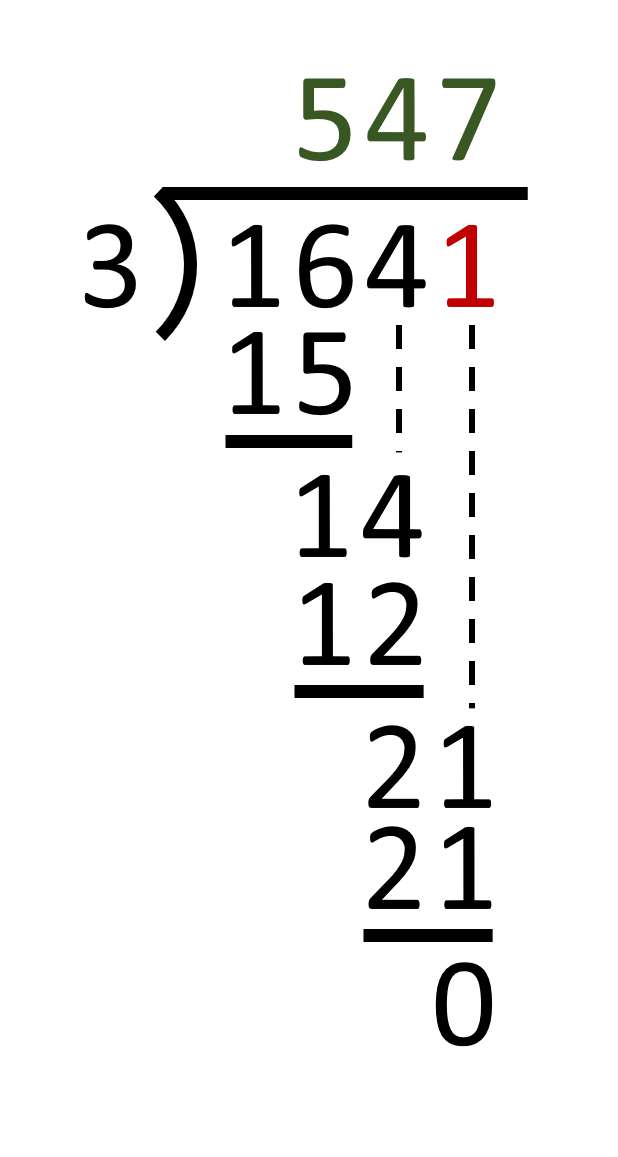# Long division calculator

## Works out the complete long division in a second.

Maximum number of decimals

## About the Long Division Calculator

The Long division calculator shows the complete work for dividing the dividend by the divisor producing the quotient. A long division is a method for dividing multidigit numbers by hand. It breaks down the division into a series of easier steps. The long division calculator shows the complete series of steps of the this method for dividing multidigit numbers. With the long division calculator you can easily check out whether the answers of your math problems are correct. You can choose if you want the long division calculator to use decimals if necessary, or just show the remainders. Doing a long division with decimals or remainders? The long division calculator helps you out. Just put the divisor in the left input field and the dividend in the right input field and click on the button "calculate long division". The Long division calculator works out the complete long division for you.

## The Long Division explained

A long division is a method for dividing multidigit numbers by hand. It breaks down the division into a series of easier steps. One number, called the dividend, is divided by another number, called the divisor, producing the result called the quotient. Read more about how to do long division

Example of a long division with no remainder (R=0):• 1641 is the dividend
• 3 is the divisor
• 547 is the quotient part of the answer
• 0 is the remainder part of the answer

The specific algorithm for long division in modern use was introduced by the English mathematician Henry Briggs (1 February 1561 – 26 January 1630). Nowadays calculators and computers have become the most common way to solve division problems. But the long division algorithm is still used for pen-and-paper division of multi-digit numbers expressed in decimal notation. It shifts gradually from the left to the right end of the dividend, subtracting the largest possible multiple of the divisor (at the digit level) at each stage. These multiples become the digits of the quotient, the final difference is called the remainder.